﻿ Impulse & Momentum | Aerospace Engineering

## Impulse & Momentum

Rocket Propulsion:

– Thrust

– Conservation of Momentum

– Impulse & Momentum

– Combustion & Exhaust Velocity

– Specific Impulse

– Rocket Engines

– Power Cycles

– Engine Cooling

– Solid Rocket Motors

– Monopropellant Engines

– Staging

.

In the preceding section we saw that Newton’s second law may be expressed in the formMultiplying both sides by dt and integrating from a time t1 to a time t2, we writeThe integral is a vector known as the linear impulse, or simply the impulse, of the force F during the time interval considered. The equation expresses that, when a particle is acted upon by a force F during a given time interval, the final momentum p2 of the particle may be obtained by adding its initial momentum p1 and the impulse of the force F during the interval of time.

When several forces act on a particle, the impulse of each of the forces must be considered. When a problem involves a system of particles, we may add vectorially the momenta of all the particles and the impulses of all the forces involved. When can then write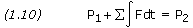For a time intervalt, we may write equation (1.10) in the form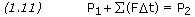Let us now see how we can apply the principle of impulse and momentum to rocket mechanics.

Consider a rocket of initial mass M which it launched vertically at time t=0. The fuel is consumed at a constant rate q and is expelled at a constant speed Ve relative to the rocket. At time t, the mass of the rocket shell and remaining fuel is M-qt, and the velocity is v. During the time intervalt, a mass of fuel qt is expelled. Denoting by u the absolute velocity of the expelled fuel, we apply the principle of impulse and momentum between timet and time t+t. Please note, this derivation neglects the effect of air resistance.We writeWe divide through byt and replace u-(v+v) with Ve, the velocity of the expelled mass relative to the rocket. Ast approaches zero, we obtainSeparating variables and integrating from t=0, v=0 to t=t, v=v, we obtain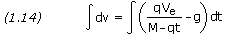which equalsThe term -gt in equation (1.15) is the result of Earth’s gravity pulling on the rocket. For a rocket drifting in space, -gt is not applicable and can be omitted. Furthermore, it is more appropriate to express the resulting velocity as a change in velocity, orV. Equation (1.15) thus becomes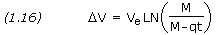Note that M represents the initial mass of the rocket and M-qt the final mass. Therefore, equation (1.16) is often written as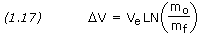where mo/mf is called the mass ratio. Equation (1.17) is also known as Tsiolkovsky’s rocket equation, named after Russian rocket pioneer Konstantin E. Tsiolkovsky (1857-1935) who first derived it.

In practical application, the variable Ve is usually replaced by the effective exhaust gas velocity, C. Equation (1.17) therefore becomes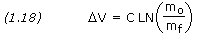Alternatively, we can writewhere e is a mathematical constant approximately equal to 2.71828.

For many spacecraft maneuvers it is necessary to calculate the duration of an engine burn required to achieve a specific change in velocity. Rearranging variables, we have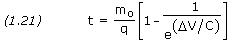—————————–

```PROBLEM 1.2

The spacecraft in problem 1.1 has an initial mass of 30,000 kg.  What is the change
in velocity if the spacecraft burns its engine for one minute?

SOLUTION,

Given:  M = 30,000 kg
q = 30 kg/s
Ve = 3,100 m/s
t = 60 s

Equation (1.16),V = Ve × LN[ M / (M - qt) ]V = 3,100 × LN[ 30,000 / (30,000 - (30 × 60)) ]V = 192 m/s```

```

PROBLEM 1.3

A spacecraft's dry mass is 75,000 kg and the effective exhaust gas velocity of its
main engine is 3,100 m/s.  How much propellant must be carried if the propulsion system
is to produce a totalv of 700 m/s?

SOLUTION,

Given:  Mf = 75,000 kg
C = 3,100 m/sV = 700 m/s

Equation (1.20),

Mo = Mf × e(DV / C)
Mo = 75,000 × e(700 / 3,100)
Mo = 94,000 kg

Propellant mass,

Mp = Mo - Mf
Mp = 94,000 - 75,000
Mp = 19,000 kg```

```

PROBLEM 1.4

A 5,000 kg spacecraft is in Earth orbit traveling at a velocity of 7,790 m/s.  Its
engine is burned to accelerate it to a velocity of 12,000 m/s placing it on an escape
trajectory.  The engine expels mass at a rate of 10 kg/s and an effective velocity of
3,000 m/s.  Calculate the duration of the burn.

SOLUTION,

Given:  M = 5,000 kg
q = 10 kg/s
C = 3,000 m/sV = 12,000 - 7,790 = 4,210 m/s

Equation (1.21),

t = M / q × [ 1 - 1 / e(DV / C) ]
t = 5,000 / 10 × [ 1 - 1 / e(4,210 / 3,000) ]
t = 377 s```